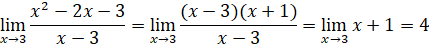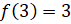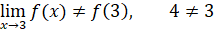# Math

Some of the content of this guide was modeled after a guide originally created by Openstax and has been adapted for the GPRC Learning Commons in October 2021. The graphs are generated using Desmos. This work is licensed under a Creative Commons BY 4.0 International License.## Continuity

A function f(x) is continuous at a number a if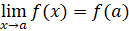This definition requires three statements to hold true for f(x) to be continuous at a:

1. f(a) is defined, or a is in the domain of f(x)
2.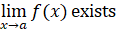, f(x) must be defined on an open interval that contains a:
3.Example 1: Discontinuous at a point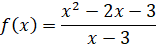This function is discontinuous at a = 3 since f(3) is not defined.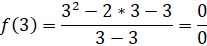Example 2: Discontinuous at the limit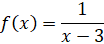This function is discontinuous at a = 3 since the limit of the function is undefined.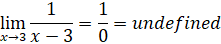Example 3: Discontinuous at the function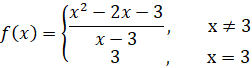This function has a discontinuity at a = 3 since the limit of the function and the value of the function are not equal.